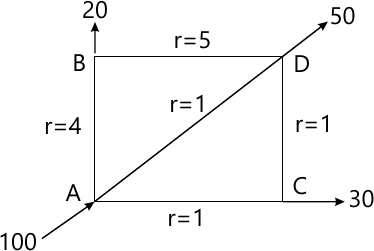MORE IN Fluid Mechanics - 2
MU Civil Engineering (Semester 4)
Fluid Mechanics - 2
December 2014
Total marks: --
Total time: --
INSTRUCTIONS
(1) Assume appropriate data and state your reasons
(2) Marks are given to the right of every question
(3) Draw neat diagrams wherever necessary

Answer any four out of the following.
1 (a) Define the terms: Major energy losses and Minor energy losses.
5 M
1 (b) Write a short note on Dash not mechanism.
5 M
1 (c) Explain water hammer with control measure.
5 M
1 (d) Define Match number and state its significance in compressible fluid flow.
5 M
1 (e) What is kinetic energy correction factor and momentum correction factor?
5 M

2 (a) A horizontal pipe-line 50m long is connected to a water tank at one end and discharge freely into the atmosphere at the other end. For the first 30m of its length from the tank, the pipe is 20mm diameter and its diameter is suddenly enlarged to 400mm. The height of water level in the tank is 10m above the center of pipe. Considering all minor losses, determine the rate of flow. Take f=0.01 for both sections of the pipe.
10 M
2 (b) Two reservation are connected by a pipe line consisting of two pipes, one of 15cm diameter and length 6m and the other of diameter 22.5cm and 16m length. If the difference of water levels in the two reservation is 6m, calculate the discharge and draw the energy gradient line. Take f=0.04.
10 M

3 (a) Prove that the velocity through the Nozzle is given by.
$V=\sqrt{\dfrac{2gH}{1+\dfrac{4fc}{D}\times \dfrac{a^{2}}{\lambda^{2}}}}$
5 M
3 (b) Solve that the pressure rise due to sudden closure of valve at the end of pipe, through which water is flowing is given by $P=\sqrt[V]{\dfrac{d}{\dfrac{1}{k}+\dfrac{D}{Et}}}$
5 M
3 (c) Calculate the discharge in each pipe of the network shown in figure below by Hardy Cross method Take n=2.10 M

4 (a) (i) Prove the following relationship for one dimensional compressible flow $\dfrac{dA}{A}=\dfrac{dV}{V}\left [ M^{2}-1 \right ]$
5 M
4 (a) (ii) Derive an expression for stagnation temperature and stagnation Density.
5 M
4 (b) (i) Find the Match number when an aero plane is flying at 900 Km/hour through still air having a pressure of 8N/cm2 and temperature -15°C. Take k=1.4 and R=287 J/kg K. Calculate the pressure, density and temperature of air at the stagnation point on the nose of plane.
8 M
4 (b) (ii) A projectile is travelling in air having pressure and temperature as 8.829 N/cm2 and 5°C. If the Match angle is 30°, find the velocity of projectile. Take k=1.4 and R=287 J/kg K.
2 M

5 (a) Derive Hagen Poiseuille Law for flow of viscous fluid of viscous fluid in circular pipes.
10 M
5 (b) A lubricating oil of viscosity 1 poise and specific gravity 0.9 is pumped through a 30mm diameter pipe. If the pressure drop per meter length of pipe is 20 KN/m2 determine.
i) The Mass flow rate in kg/min
ii) The shear stress at the pipe wall,
iii) The Reynolds number of flow , and
iv) The power required per 50m length of the pipe to maintain the flow.
10 M

6 (a) Obtain an expression for velocity distribution for turbulent flow in smooth pipes.
10 M
6 (b) For turbulent flow in a pipe of diameter 300mm, find the discharge when the center line velocity is 2.0 m/s and the velocity at a point 100 mm from the center as measured by pitot tube is 1.6 m/s.
10 M

More question papers from Fluid Mechanics - 2